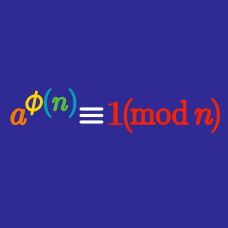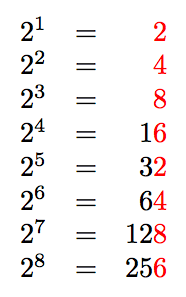Number Theory

# Euler's Theorem: Level 2 ChallengesWhat is the last digit of $2^9?$

For a positive integer $n>2,$ what can we say about the number of positive integers less than $n$ that are relatively prime to $n$ (that is, their GCD is 1)?

Hint: A useful property of the Greatest Common Divisor function is $\gcd(a,b) = \gcd(a,a-b);$ e.g., $\gcd(10,8) = \gcd(10,2) = 2.$

Find the last two digits of $7^{100}-3^{100}.$

What is the largest integer that always divides $n^{7}-n$ for any integer $n?$

$\LARGE \color{#3D99F6}5^{\color{#20A900}5^{\color{#D61F06}5^{\color{#624F41}5^\color{magenta}5}}}$

What are the last three digits of the number above?

×